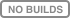`nevergrad` is a Python 3.6+ library. It can be installed with:

``pip install nevergrad``

More installation options, including windows installation, and complete instructions are available in the "Getting started" section of the documentation.

Minimizing a function using an optimizer (here `NGO`) is straightforward:

``````import nevergrad as ng

def square(x):
return sum((x - .5)**2)

optimizer = ng.optimizers.NGO(parametrization=2, budget=100)
recommendation = optimizer.minimize(square)
print(recommendation.value)  # recommended value
>>> [0.49971112 0.5002944]``````

`nevergrad` can also support bounded continuous variables as well as discrete variables, and mixture of those. To do this, one can specify the input space:

``````import nevergrad as ng

def fake_training(learning_rate: float, batch_size: int, architecture: str) -> float:
# optimal for learning_rate=0.2, batch_size=4, architecture="conv"
return (learning_rate - 0.2)**2 + (batch_size - 4)**2 + (0 if architecture == "conv" else 10)

# Instrumentation class is used for functions with multiple inputs
# (positional and/or keywords)
parametrization = ng.p.Instrumentation(
# a log-distributed scalar between 0.001 and 1.0
learning_rate=ng.p.Log(lower=0.001, upper=1.0),
# an integer from 1 to 12
batch_size=ng.p.Scalar(lower=1, upper=12).set_integer_casting(),
# either "conv" or "fc"
architecture=ng.p.Choice(["conv", "fc"])
)

optimizer = ng.optimizers.NGO(parametrization=parametrization, budget=100)
recommendation = optimizer.minimize(fake_training)

# show the recommended keyword arguments of the function
print(recommendation.kwargs)
>>> {'learning_rate': 0.1998, 'batch_size': 4, 'architecture': 'conv'}``````Convergence of a population of points to the minima with two-points DE.

## Documentation

Check out our documentation! It's still a work in progress, don't hesitate to submit issues and/or PR to update it and make it clearer!

## Citing

``````@misc{nevergrad,
author = {J. Rapin and O. Teytaud},
`nevergrad` is released under the MIT license. See LICENSE for additional details about it.